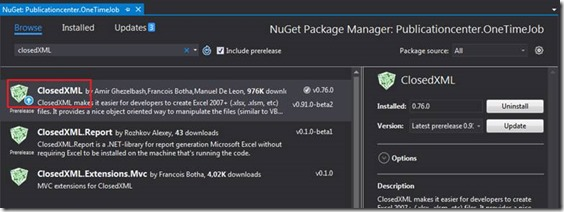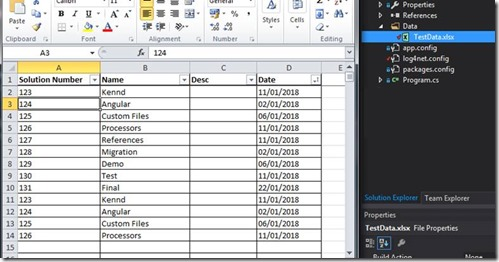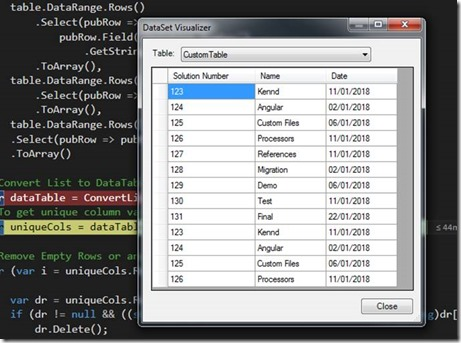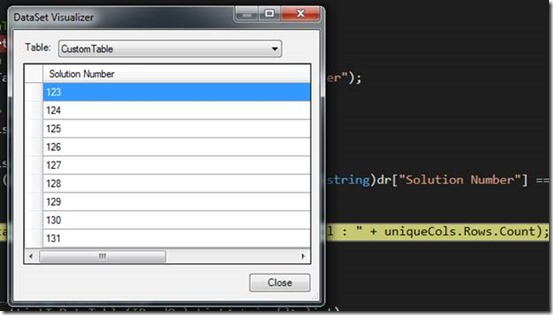# How to get Data from Excel to DataTable using ClosedXML in C# and avoid duplicate rowsSenior Developer
January 21, 2018

[Total: 1    Average: 5/5]

Views
12861

Hello everyone,

We had a requirement to read data from Excel and get it in your code. We utilized ClosedXML to achieve this functionality. ClosedXML is one of the useful dll which is used for accessing Excel, XML files and manipulate those files, to know more about ClosedXML – please refer this github. In this article we will see how to easily fetch data from Excel by column names.

Add ClosedXML reference to your project, by going to Manage NuGet Package, as shown below.As you can see in the below screenshot, we have TestData Excel file in Data folder.Initially we are getting data from Excel and querying the data based on column name and pass it to a List<string[]> then we are converting it to DataTable using a function ConvertListToDataTable based on your requirement.

``` private void GetDataFromExcel()
{
var xmlFile = Path.Combine(Environment.CurrentDirectory, "Data\\TestData.xlsx");
using (var workBook = new XLWorkbook(xmlFile))
{
var workSheet = workBook.Worksheet(1);
var firstRowUsed = workSheet.FirstRowUsed();

// Get a range with the remainder of the worksheet data (the range used)
// Treat the range as a table (to be able to use the column names)
var table = range.AsTable();

//Specify what are all the Columns you need to get from Excel
var dataList = new List<string[]>
{
table.DataRange.Rows()
.Select(tableRow =>
tableRow.Field("Solution Number")
.GetString())
.ToArray(),
table.DataRange.Rows()
.Select(tableRow => tableRow.Field("Name").GetString())
.ToArray(),
table.DataRange.Rows()
.Select(tableRow => tableRow.Field("Date").GetString())
.ToArray()
};
//Convert List to DataTable
var dataTable = ConvertListToDataTable(dataList);
//To get unique column values, to avoid duplication
var uniqueCols = dataTable.DefaultView.ToTable(true, "Solution Number");

//Remove Empty Rows or any specify rows as per your requirement
for (var i = uniqueCols.Rows.Count - 1; i >= 0; i--)
{
var dr = uniqueCols.Rows[i];
if (dr != null && ((string)dr["Solution Number"] == "None" || (string)dr["Title"] == ""))
dr.Delete();
}
Console.WriteLine("Total number of unique solution number in Excel : " + uniqueCols.Rows.Count);
}
}

private static DataTable ConvertListToDataTable(IReadOnlyList<string[]> list)
{
var table = new DataTable("CustomTable");
var rows = list.Select(array => array.Length).Concat(new[] { 0 }).Max();

for (var j = 0; j < rows; j++)
{
var row = table.NewRow();
row["Solution Number"] = list[j];
row["Name"] = list[j];
row["Date"] = list[j];
}
return table;
}

```

In the below screenshot you can find all the data in the DataTable.UniqueCols holds the unique rows in ‘Solution Number’ column,The reason for getting unique value is that, you can query main DataTable by using this unique value so that you can get all the rows with this particular value.

Happy Coding

Ahamed

### Author Info